# Use of omega(Ω,ω) symbols with help of LaTeX

Omega(Ω) is an important symbol for scientific use. If you want to make a beautiful document, you need to know many uses of this symbol.

Different latex syntax of this symbol is given in the table below.

\documentclass{article}
\begin{document}
$$[S] = [\Omega^{-1}]$$
$$\Omega = -K_B T\log Z$$
$$\Omega = \frac{A}{r^2}$$
$$\Omega \equiv \frac{\rho}{\rho_c}$$
$$\Omega = \{S_1, S_2, \dots, S_n\}$$
\end{document}

Output :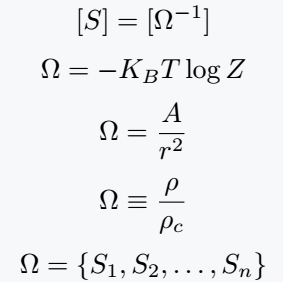Best practice is to use \omega command for Capital Omega symbol. And below are some more complex expressions that will help make your document more beautiful.

\documentclass{article}
\begin{document}
$$\mathrm{d}\Omega = \sin\theta d\theta d\phi$$
$$\frac{\partial \Omega}{\partial \phi} = 4\pi\sin^{2}\frac{\theta}{2}d\theta$$
$$\sum_{i=1}^n \Omega_i = 2\sum_{i=1}^{n-2}-4\pi$$
$$\Omega_d = \frac{2\pi^{\frac{d}{2}}}{t}$$
\end{document}

Output :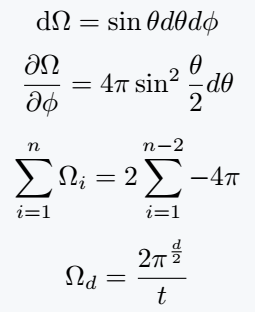## Use superscript and subscript with big omega

If you want to use Superscript and Subscript with a symbol, you have to use both expressions at the same time.

I think the most beautiful use of latex is that you can define new commands with different arguments.

\documentclass{article}
\newcommand\Ome{\Omega^{#1}_{#2}}
\newcommand\Omex{\Omega_{#1}}
\begin{document}
$$\Ome{0}{c} \quad \Ome{-}{b}$$
$$\Ome{+}{cc} \quad \Ome{0}{cb}$$
$$\Ome{0}{cbb} \quad \Ome{-}{bbb}$$
$$\Ome{+}{cc} \quad \Ome{0}{cb}$$
$$\Omex{c}(3050)^0 \quad \Omex{c}(3119)^0$$
$$\Omex{0,R} \quad \Omex{0,M}$$
$$\Omega = e^{-\Omega}$$
\end{document}

Output :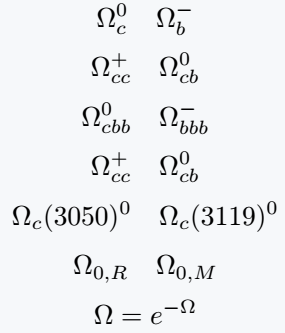Two arguments are defined for superscript and subscript with \Omex command.

## Use mho symbol in LaTeX

If you reverse the Big Omega symbol, you will get the mho symbol. Older versions of Latex defined the \mho command for this symbol, which is not currently supported.

Of course, the exact same command you have in more different packages like amssymb, stix, etc.

\documentclass{article}
\usepackage{amssymb}
\begin{document}
$$\mho^{(i)}(G) = \left\{g^{p^i} : g^{\epsilon^G} \right\}$$
$$\mho(H) = \Omega(G)$$
$$G=\mho^0(G) \geq \mho^1(G) \geq \dots \geq \mho^{K-1}(G) > \mho^k (G)$$
$$\mho^i (G/N) \blacktriangleleft G$$
$$\left|\mho^i(G).\mho^{i+1}(G)\right|$$
\end{document}

Output :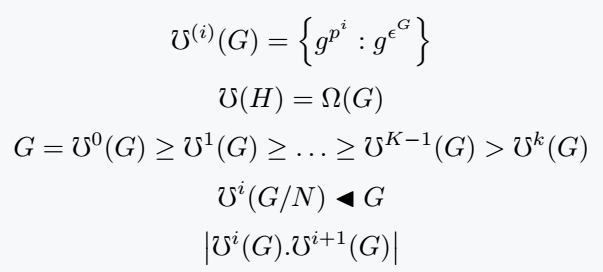## Use bold omega symbol as vector in LaTeX

Omega commands should be used for small omega symbols. This symbol is used to represent vector quantity in physics.

A vector quantity is denoted by text-style bold and placing a vector mark on the symbol.

\documentclass{article}
\usepackage{amsmath}
\begin{document}
$$\boldsymbol{\omega} = \omega\mathbf{n}$$
$$\boldsymbol{\omega} = \frac{\mathbf{r}\times\mathbf{v}}{r^2}$$
$$\vec{v}_\perp = \vec{w} + \vec{r}$$
\end{document}

Output :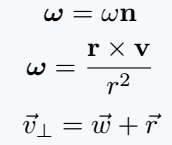#### Md Jidan Mondal

LaTeX expert with over 10 years of experience in document preparation and typesetting. Specializes in creating professional documents, reports, and presentations using LaTeX.